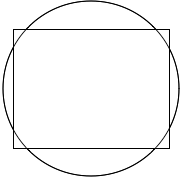SEARCH HOMEMath Central Quandaries & QueriesQuestion from christine, a parent: will an 18ft round pool fit on a 12x16 ft rectangular cement slab?Hello Christine,
We can find the length of the diagonal for the concrete slab using the Pythagorean Theorem:

122 + 162 = d2

From which we get d = 20 feet. So, the 18 ft diameter pool will “fit” on the slab.  Here is a picture:The ratios of these shapes match your situation, so this is how it will look.  Notice the substantial overhang on the top and bottom (3 ft).  I assume that this pool will be put to practical use so I will provide a few words of caution:

The area covered by one of these overhangs is about 27.9 square feet.  Therefore if the pool is to filled with say 5 feet of water, then there will be 139.5 cubic feet of water not resting on the slab.  Water weighs about 62.4 pounds per cubic foot, thus there will be roughly 8700 pounds of water in the overhang.
Each of the side overhangs will contain about 1750 pounds of water, which is still a significant amount.
Keep these things in mind so that the 9500 gallons of water remains in your pool and not in your yard.

Have fun and enjoy your summer!

TylerMath Central is supported by the University of Regina and The Pacific Institute for the Mathematical Sciences.